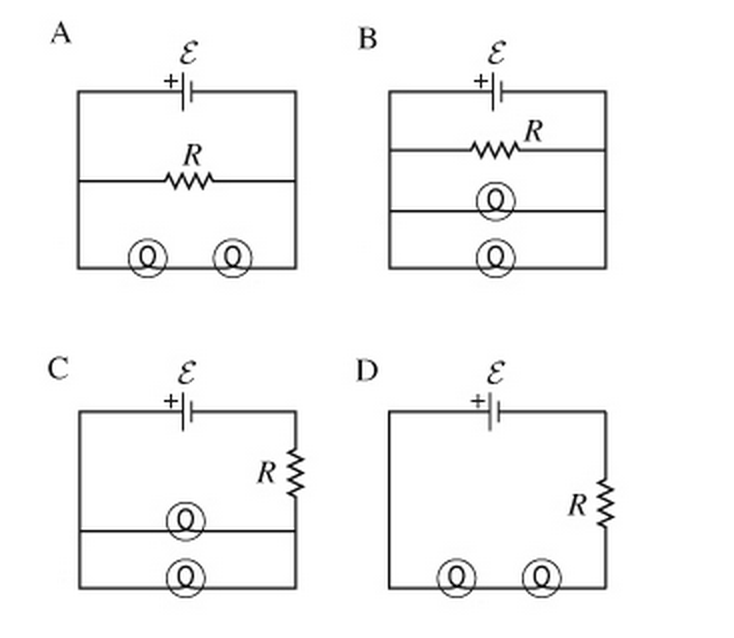# Problem: Two bulbs are connected in parallel across a source of emf E = 12.0 V with negligible internal resistance. One bulb has a resistance of 3.0 Ω , and the other is 4.0 Ω. A resistor R is connected in the circuit in series with the two bulbs.(A)Which of the following circuit diagrams represents the circuit described in this problem?(B)Which of the following statements are correct for the circuit described in this problem? Check all that apply.a. The current flowing through each bulb will be the same, regardless of their different individual resistances.b. The total current flowing through the two-bulb combination equals the passing through resistor R.c. The voltage across each bulb will be the same, regardless of their different individual resistances.d. Each bulb will have a different voltage, which will depend upon its individual resistance.

###### FREE Expert Solution

(A)

If two bulbs are connected in parallel across a source and a resistor R connected in the circuit in series with the two bulbs, we will have:

81% (96 ratings)###### Problem Details

Two bulbs are connected in parallel across a source of emf E = 12.0 V with negligible internal resistance. One bulb has a resistance of 3.0 Ω , and the other is 4.0 Ω. A resistor R is connected in the circuit in series with the two bulbs.

(A)Which of the following circuit diagrams represents the circuit described in this problem?(B)Which of the following statements are correct for the circuit described in this problem? Check all that apply.
a. The current flowing through each bulb will be the same, regardless of their different individual resistances.
b. The total current flowing through the two-bulb combination equals the passing through resistor R.
c. The voltage across each bulb will be the same, regardless of their different individual resistances.
d. Each bulb will have a different voltage, which will depend upon its individual resistance.